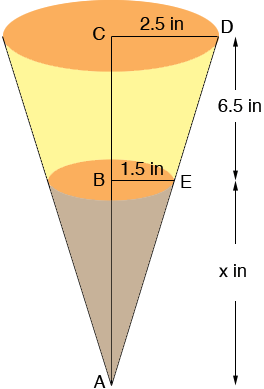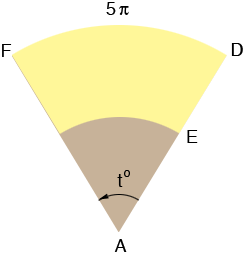SEARCH HOMEMath Central Quandaries & QueriesHello, I have attempted to use two of your answers already given and had no real success. This young lady is making a cat food dispenser using a truncated cone. The top of the cone as a diameter of 5 inches with a height of 6 1/2 inches and diameter of 3 inches for the bottom. Could you please map out a solution for the both of us to understand? Thank you so much for your time and for this wonderful service. RussellHi Russell,

One measurement in a cone or truncated cone that can be confusing is the height. I took your measurement, 6.5 inches, as the vertical height rather than the slant height. In my diagram below the distance from E to D is the slant height of the truncated cone and the distance from A to D is the slant height of the cone.I extended d the sides of the cat food dispenser to the point A in the diagram. The length x inches is the distance from B to A. I want to find the length AD and I do it by using similar triangles and Pythagoras' theorem. (Russell you used mixed fraction notation in your question but I am using decimal fractions simple because they are easier to type.)

Triangles DCA and EBA are similar so

(x + 6.5)/2.5 = x/1.5

thus

1.5 x + 9.75 = 2.5 x, or x = 9.75 inches.

Hence the length of AC is 9.75 + 6.5 = 16.25 inches.

Triangle DCA is a right triangle so, using Pythagoras' theorem

16.252 + 2.5 2 = |AD|2

In a similar fashion I found that |AE| = 9.86 inches.

Now slice along the line segment AD and roll the cone out flat to form a segment of the circle of radius(These diagrams are not to scale.)

All that remains is to find the measure of the angle at the centre of the sector, that is to in the diagram.

The length of the arc DF is the circumference of the circle at the top of the cat food dispenser, that is a circle of radius
2.5 inches. Thus the length of the arc DF is 2r = 5inches. This length is a fraction of the circumference of the circle of radius |AD| = 16.44 inches, which is 216.44 = 32.88inches. The angle measure to is also a fraction of the angle at the centre of the complete circle, that is 360o. In fact these fractions are the same, that is

(5)/(32.88) = t/360

Thus

t = (5/32.88)360 = 54.7 degrees.

I hope this helps,
PennyMath Central is supported by the University of Regina and The Pacific Institute for the Mathematical Sciences.